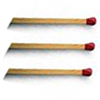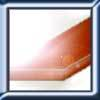#### You may also like### Construct-o-straws

Make a cube out of straws and have a go at this practical challenge.### Matchsticks

Reasoning about the number of matches needed to build squares that share their sides.### Little Boxes

How many different cuboids can you make when you use four CDs or DVDs? How about using five, then six?

# Child's Play

##### Age 7 to 11Challenge Level

Thomas and Daniel have sent this almost complete solution. Well done.

I have omitted a part of the solution where they talked about the icosahedron being able to fit into three triangular hollows. I think they were trying to say that three of the five platonic solids have triangular faces so the toy would have three triangular hollows. This would mean that the triangle could fit the toy $3 \times 12$ ways, the octahedron $3 \times 24$ ways and the isocahedron $3 \times 60$ ways - that complicates things a little, but then of course there are the number of ways you can arrange the three solids with triangular faces on the toy (would that matter?). So the number of times the bell rings wasn't quite correct but I think this part of the question is open to some interpretation anyway.

The tetrahedron can fit into the triangular hollow twelve different ways as it has four sides and for each side three different ways of fitting in.

The cube having six sides in the shape of squares can fit into the square hollow in four different ways and so fits twenty-four ways.

The octahedron has eight sides in triangles and so fits in twenty-four different ways.

The dodecahedron with twelve sides in pentatonic shapes fits sixty times.

The icosahedron can fit into the triangular hollow sixty times.

Add all the ways that each fits into the suited hollow and you find the number of times the bell rings.

Andy emailed us and suggested that there could be $24$ ways of fitting the tetrahdron into the hollows if you could put the tetrahedron in "vertex first". This might be possible if the hollow was deep enough. Thank you for drawing this to our attention, Andy.